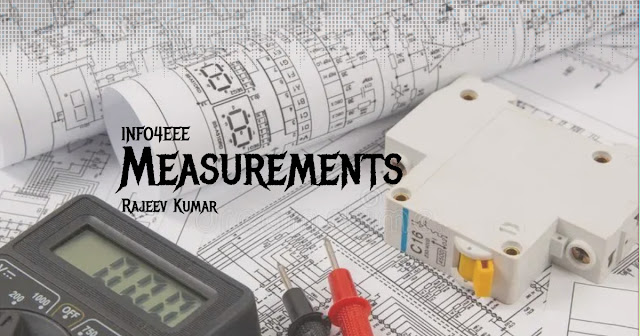## Permanent Magnet Moving Coil Instruments (PMMC)

The permanent magnet moving coil instruments are most accurate type for d.c. measurements. The action of these instruments is based on principle of motor. It consists of permanent magnet with soft iron (B). A cylindrical iron core is mounted between two poles of magnet giving very narrow air gap to rectangular coil. The rectangular coil is wounded by many turns of aluminium on copper wire acting as moving elements. The purpose of using core is to make the field uniform and reduce reluctance of magnetic circuits. A low reluctance helps to retain the permanence of the magnet.

If i is the current flowing through the coil of N turns and length I meters and B is flux density. Then deflecting force,
F = BiAN netwons

Where, F =  Deflection force (Nm), B= flux density in airgap Wb/meter srqaure, N= Number of turns in coil, I= current in moving coil, A=effective coil area

When a current carrying coil is placed in the magnetic field produced by permanent. magnet, the coil experiences a force and moves. As the coil is moving and the magnet permanent, the instrument is called permanent magnet moving coil instrument. This basic principle is called D'Arsonval principle. The amount of force experienced by Scale Mirror the coil is proportional to the current passing through the coil. N S

The moving coil is either rectangular or circular in shape. It has number of turns of fine wire. The coil is suspended so that it is free to turn about its vertical axis. The coil is placed in a uniform, horizontal and radial magnetic field of a permanent magnet in the shape of a horse-shoe. The iron core is spherical if coil is circular and is cylindrical if the coil is rectangular. Due to iron core, the deflecting torque increases, increasing the sensitivity of the instrument.

The controlling torque is provided by two phosphor bronze hairsprings. The damping torque is provided by eddy current damping. It is obtained by movement of the aluminium former, moving in the magnetic field of the permanent magnet.

The pointer is carried by the spindle and it moves over a graduated scale. The pointer has lightweight so that it can deflect rapidly. The mirror is placed below the pointer to get an accurate reading by removing the parallax. The weight of the instrument is normally counterbalanced by the weights situated diametrically opposite and rigidly connected to it. The scale markings of the basic d.c. PMMC instruments are usually linearly spaced as the deflecting torque and hence the pointer deflection are directly proportional to the current passing through the coil.

The various advantages of PMMC instruments are,
1. It has a uniform scale.
2. With a powerful magnet, its torque to weight ratio is very high. So operating current is small.
3. The sensitivity is high.
4. The eddy currents induced in the metallic former over which coil is wound, provide effective damping  It consumes low power, of the order of 25 W to 200 μW.
5. It has high accuracy.
6. Instrument is free from hysteresis error.
7. Extension of instrument range is possible.
8. Not affected by external magnetic fields called stray magnetic fields.